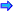# Binary Operators - Arithmetic

There are times when it is desirable to extend the binary arithmetic operators: +, -, /, * and %. These operators as with all operators may be members or non members. The relationship between the syntax and the call is shown in the table below:Open table as spreadsheet

Is Member?

Code Written

Actual Call

YES

A OP B

A.OP(B)

NO

A OP B

OP(A,B)where OP is the binary operator and A is a class object in the first case but not in the second case.

Not every class lends itself to all operators. The class Date discussed earlier works fine for each of the unary operators but it has no need for all of the binary operators when the operands are two class objects.

A class for which most of the binary operators on objects would be meaningful would be the complex numbers. For example: complex.cpp. While it is possible to write:` cComplex = aComplex + bComplex; `the actual code for this member function is:` cComplex = aComplex.+(bComplex); `While not all binary operators would be meaningful on the class Date, some would. For example:` 10/21/2007 - 9/12/2006 `would be meaningful to find the number of days between two Dates. Notice that this would return an integer and not a Date.

Other useful binary operations on the class Date would be the following:` 10/21/2007 + 10 `or` 10/21/2007 – 10 `These would yield the Dates 10 days in the future or in the past respectively. In these examples not all operands nor the return values are required to be class objects. For example: dateoptr.cpp.

These examples of binary operators will still preserve the order of operator precedence that the syntax requires. However from your mathematics classes, you have learned additional rules like the law of communitivity. While this rule works fine for the Complex class, it does not work for the Date class. For example:` 10/21/2007 + 10 `is not equivalent to` 10 + 10/21/2007 `Even though this may seem reasonable, they will not be equivalent unless a friend function is used. This is necessary because member operator functions must have the first operand be a class object. As you can observe, 10 is not a class object of the class Date. This non-member operation is possible with a prototype like the following in the class definition:` Date friend operator +(long numberDays, Date aDate); `The body of the function will be the same except any reference to the private data member must be dotted with the object aDate since no object will be calling the function. See example datoptr2.cpp.